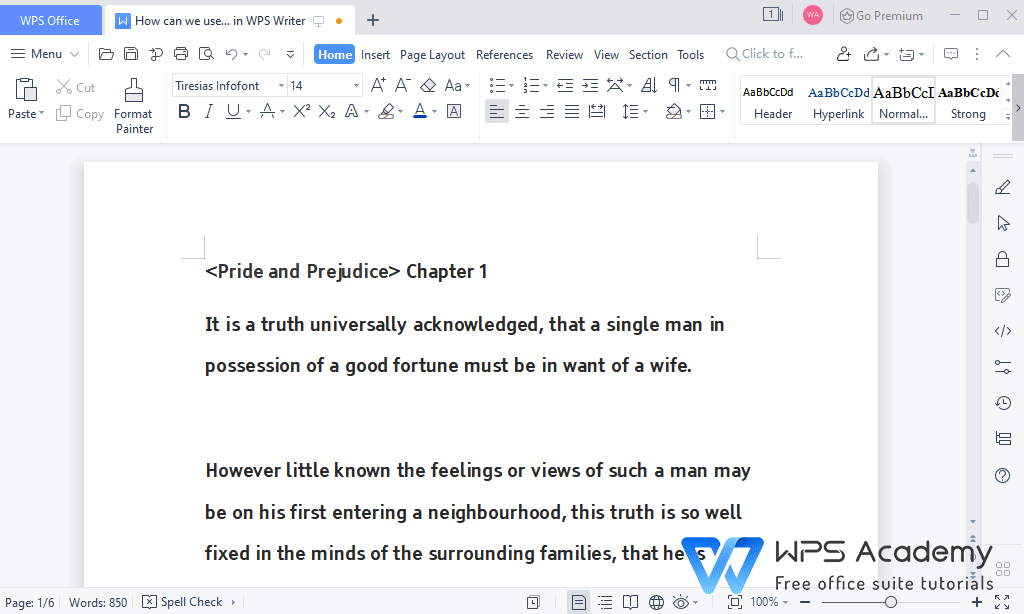# How can we use equation editor in WPS Writer

September 28, 2021
9,060 Views

1. Open the document, click Insert Equation Equation Editor.

2. In the Equation Editor, the menu bar is on the top, and some commonly used formula commands are below the menu bar.

3. When we place the mouse cursor on the formula commands, we can see the corresponding symbol elements, etc.

So, we can write the formula according to our needs.

4. After writing, we can click File  Update in the upper left corner. At the moment ,the formula has been inserted into the document.

5. If we need to beautify the formula, we can select the formula and click Picture Tools to adjust it.Perfect your work with WPS Writer like Microsoft word.